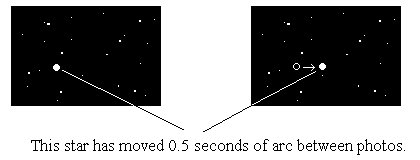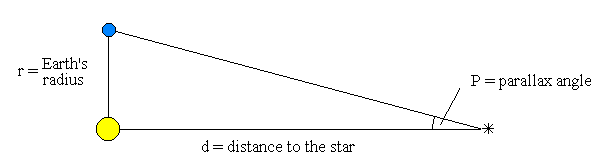# How the calculate the distance

For the star in Figure 1, the parallax angle - P is half the distance moved by the star between photos. Therefore
P = 0.5 / 2 = 0.25 seconds of arc. (1 second of arc (1") = 1 / 3600) degrees

Figure 1From Figure 2, the distance between the Sun and the star is :
d = r / tan P
If P is 1 second of arc:
d = 150 000 000 / tan 1" = 30 million million km
This distance is called one parsec and is a basic unit for measuring astronomical distances.
Distance in parsecs = 1 / P in seconds of arcFigure 2

For the star in Figure 1:
d = 1 / P = 1 / 0.25 = 4
Therefore the star is four parsecs away.